Create a new printableRatios
Math Worksheets

Sample - Click above to make a new math worksheet (PDF).
 Name _____________________________Date ___________________
Ratios
Use a proportion to find the unknown length in the pair of similar figures.
(drawings are not drawn to scale)

1.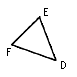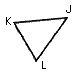length of sides:
 FD  = 104 m ED  = ________ FE  = 24 m

 KL  = 208 m JL  = 176 m KJ  = 48 m
2.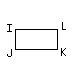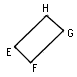length of sides:
 JK  = 16 yd LI  = 16 yd IJ  = 56 yd KL  = 56 yd

 EF  = 21 yd FG  = 6 yd GH  = 21 yd HE  = ________
3.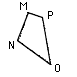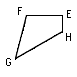length of sides:
 MN  = 6 km PM  = ________ OP  = 8 km NO  = 4 km

 HG  = 64 km GF  = 40 km EH  = 32 km FE  = 48 km
4.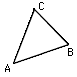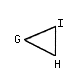length of sides:
 AB  = 96 cm AC  = 72 cm CB  = 80 cm

 GH  = 72 cm IH  = ________ GI  = 54 cm
 5 * This is a pre-made sheet.Use the link at the top of the page for a printable page.
6.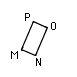length of sides:
 IJ  = 21 in LI  = 42 in JK  = 42 in KL  = 21 in

 NO  = 48 in PM  = ________ OP  = 24 in MN  = 24 in